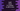# Swift program to check if a number is odd or even# Find if a number is even or odd in swift :

A number is even if it is divisible by 2, else it is odd. If you want to check if a number is odd or even, you can simply use modulo % operator in swift. We can also find out the remainder by using isMultiple and remainder methods. In this post, I will show you how to iterate through the elements of an integer array and how to check odd/even for each number in that array.

## Using the modulo operator :

Modulo operator returns the remainder dividing one number by a different number.

``````var arr = [1,2,3,4,5,6]

for n in arr{
if(n % 2 == 0){
print("\(n) is even")
}else{
print("\(n) is odd")
}
}``````

### Output :

``````1 is odd
2 is even
3 is odd
4 is even
5 is odd
6 is even``````

## Using isMultiple :

isMultiple(of:) is an instance method that returns one boolean value if a number is multiple of another number.true if the number is multiple of another number, else false.

``````var arr = [1,2,3,4,5,6]

for n in arr{
if(n.isMultiple(of: 2)){
print("\(n) is even")
}else{
print("\(n) is odd")
}
}``````

It prints the same output.

## For double :

Swift double comes with one instance method to find out the remainder :

``remainder(dividingBy:)``

It divides a number by a different number and returns the remainder.

``````var arr:[Double] = [1,2,3,4,5,6]

for n in arr{
if(n.remainder(dividingBy: 2) == 0){
print("\(n) is even")
}else{
print("\(n) is odd")
}
}``````

### Output :

``````1.0 is odd
2.0 is even
3.0 is odd
4.0 is even
5.0 is odd
6.0 is even``````